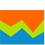# 100% stacked area chart100% stacked area chart

A 100% stacked area chart shows two or more measures across one dimension. This chart type displays measures placed on top of each other. Each measure is represented by a different color. The height of each colored stack represents the percentage proportion of that measure related to the whole. The sum of all the measures represents 100% of a dimension. The y-axis scale is always 100%.

This chart is best used to:

• Highlight the percentage that each measure contributes to a dimension when the cumulative total is not important.
• Show trends among measures over time, or across another dimension.

## When to use

Use the 100% stacked area chart to illustrate the percentage that each of the following measures contributes to 100% of a dimension:

• Sales and marketing costs, travel expenses, and entertainment costs – to the operating cost for each department.
• Building rent, insurance costs, subscriptions, and utilities – to the administrative expenses for each city.
• Operating and non-operating costs – to the total costs for each line of business and country.

## Data requirements

To build this chart, define the data fields as follows:

• Dimension – One dimension
• Measure – Two or more measures
• Tooltip – (Optional) One or more measures
• Trellis – (Optional) One dimension

## Use case

The following chart shows the percentage contributed by each of the following measures to the operating cost over a year:

In this chart, the sum of all the measures represents 100% of the operating cost.

Also, the chart shows the trend for the three measures. By looking at the colors, you can determine which color areas increase or decrease in height as they move along the time axis.

## References

For details on how to customize your visualization, see Visualization settings.

For a whole list of visualizations, see the following topics: